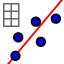# IM1.8.5 CC 2

Author:
Lind, Jon

## Apply

Think you understand the CC? Put the following scatterplots in order (left to right) of increasing CC

1. Write the letters of the data sets in order of increasing CC

## Calculating the CC

In the spreadsheet below is the actual data from the sets above. Calculate the CC for each set and write it in the orange boxes. To find the CC: 1. Select both columns of numbers (just the numbers, not the whole column), and choose Two Variable Regression Analysis. This should make a scatter plot of your data. 2. 2. Press the Show Statistics button, and find the CC (do you remember what variable is used for the CC? Check the last activity if you don't). 3. Write your CC in the orange box, and move on to the next set of data.

2. Was your answer to #1 correct? Explain how to estimate the CC based on a scatter plot.